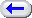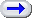Emacs \$B\$i\$/\$i\$/F~Lg(B \$B\$J\$+8+(B! \$B8!:w(B     \$B4D6-(B \$B9=@.(B \$B@aL\   \$BL\ \$BL\ \$BL\ \$BL\ \$B%3%i%`L\ \$B@58mI=(B \$B;2>H(B   \$BK\J8Fb(B URL anoncvs \$BK\J8JdB-(B p142 \$B@55,I=8=(B p145 c \$B\$H\$\$\$&7A<0\$N(B       \$B\$^\$H\$a(B p145 \$BJ,N`(B p185 (outline-mode) p208 Wanderlust/Meadow p252 (POP-before..) p252 (SMTPauth) p257 (draft) p262 (Relay) p286 AucTex p287 auto-insert p303 (ssh) p351 .Xdefaults p399 .emacs(Mac OS X) p400 \$B\$3\$H\$(\$j(B patch       \$B66K\\$5\$s\$+\$i(B p400 inline update       mule-ucs       bash \$B\$H(B tcsh \$B\$NFIBX(B p400 inline update       mule-ucs       bash \$B\$H(B tcsh \$B\$NFIBX(B p400 20060319 \$BHG(B       ~/.emacs \$B\$N@_Dj(B       inline \$B\$rM-8z\$K(B       transparency \$B\$N@_Dj(B       bash \$B\$H(B tcsh \$B\$NFIBX(B Carbon package p401 tamago p411 emacs-w3m p412 auctex p413 mu-cite p415 x-face p425 FreeWnn (FC3)       inetd       \$B<+F05/F0(B p435 w3m \$B=q\$1\$J\$+\$C\$?\$3\$H(B   grep     egrep Anthy Meadow   Windows \$B\$G(B Emacs     setup.exe ImageMagick HOME .emacs \$B\$=\$NB>(B   dot.emacs \$B=PHG rank \$B4XO"=q@R(BEmacs \$B\$N;O\$aJ}(B | dot.emacs | application | \$BJXMx\$J%D!<%k(B Emacs \$B\$i\$/\$i\$/F~Lg(B
Last Update: "2006/03/09 07:45:09 makoto"

#### p145 \$BJ,N`(B

\$B@55,I=8=(B(\$BI=(B3.27, p145) \$B\$NCf\$K(B Category (\$BJ,N`(B)\$B\$,H4\$1\$F\$\$\$^\$7\$?!#(B
 \cC C \$B\$H\$\$\$&J,N`\$NJ8;z\$K0lCW\$9\$k(B \CC C \$B\$H\$\$\$&J,N`(B\$B0J30(B\$B\$NJ8;z\$K0lCW\$9\$k(B
\$B>e\$NI=\$G\$O(B C \$B\$H\$\$\$&J8\$O \$BJ,N`J8;z@55,I=8=\$NNc(B:
 \cc \$BCfJ8J8;z(B \cg \$B%.%j%7%cJ8;z(B \ck \$BF|K\8l(B 1 \$B%P%\$%H%+%?%+%J(B
\$B8=:_\$N%b!<%I\$G\$O!"\$I\$N\$h\$&\$JJ,N`J8;z\$,;H\$(\$k\$+\$O(B
```M-x describe-categories
```
\$B\$GI=<(\$5\$l\$^\$9!#\$=\$NI=<(\$N:G8e\$NJ}\$K\$O!" \$BJ,N`J8;z@55,I=8=\$K;H\$(\$kJ8;z\$NNc(B
 0 consonant 1 base (independent) vowel 2 upper diacritical mark (including upper vowel) 3 lower diacritical mark (including lower vowel) 4 tone mark 5 symbol 6 digit 7 vowel-modifying diacritical mark 8 vowel-signs 9 semivowel lower < A character which can't be placed at end of line. > A character which can't be placed at beginning of line. @ invalid japanese char category A Alpha-numeric characters of 2-byte character sets C Chinese (Han) characters of 2-byte character sets G Greek characters of 2-byte character sets H Japanese Hiragana characters of 2-byte character sets \$BJ?2>L>(B I Indian Glyphs K Japanese Katakana characters of 2-byte character sets \$BJR2>L>(B N Korean Hangul characters of 2-byte character sets Y Cyrillic characters of 2-byte character sets ^ Combining diacritic or mark a ASCII b Arabic c Chinese e Ethiopic (Ge'ez) g Greek h Korean i Indian j Japanese \$BA4\$F\$NF|K\8lJ8;z(B k Japanese katakana 1 \$B%P%\$%HJR2>L>(B l Latin o Lao q Tibetan r Japanese roman t Thai v Vietnamese w Hebrew y Cyrillic | While filling, we can break a line at this character.
\$BNc\$(\$P(B \ck \$B\$H\$9\$k\$H!">e\$NI=\$N(B \$B!V(BJapanese katakana\$B!W\$N9`L\\$K\$J\$jH>3Q%+%?%+%J\$NJ8;z\$K0lCW\$7\$^\$9!#(B
 Last Update: Sat, 07 Jun 2014 22:16:17 GMT